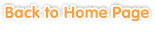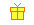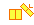Sample questions are shown below. Just click to start!Exercises >> Grade 1

• Chapter 1
From 0 to 10
••
Comparing Numbers and Sequencing••
Subtraction•• Chapter 2
Numbers 11 to 20
•
Counting•
Comparing Numbers and Sequencing••
Addition Up to 20 without Regrouping•
Addition Up to 20 with Regrouping•
Subtraction Up to 20 without Regrouping•
Subtraction Up to 20 with Regrouping•• Chapter 3
Problem Solving: Numbers up to 20• Chapter 4
Numbers 20 to 100
•
Counting by Groups••
Place Value and Writing Numbers•
Ordinal Numbers•
Adding and Subtracting 10s•
Adding 2-Digit Numbers with 10s•
Adding 2-Digit Numbers with 1-Digit Numbers•
Subtracting 2-Digit Numbers with 10s•
Subtracting 2-Digit Numbers with 1-Digit Numbers•• Chapter 5• Chapter 6• Chapter 7
Patterns
••• Chapter 8
Classifying• Chapter 9• Chapter 10
Telling Time to Hour, Half Hour, and Quarter Hour• Chapter 11
Money
••
Problem Solving• Chapter 12
Reading Graphs and Tables